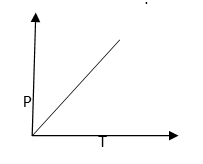# Gay Lussacs Law Formula

Gay Lussac’s law defines the relationship between the pressure and temperature of a gas. According to the law, when volume is held constant, the pressure of an ideal gas is directly proportional to its temperature. This law can be expressed as follows:

$\frac{P_{1}}{T_{1}}=\frac{P_{2}}{T_{2}}$

Where, P1 and P2 are the Initial Pressure and Final Pressure respectively. Both the pressures are in the units of atm. T1 refers to the Initial Temperature and T2 refers to the Final Temperature. The temperatures are measured in the units of Kelvin.

This can be explained as, at constant volume of a close container, if the pressure inside container increases, the temperature inside the container also increases. Pressure vs. Temperature graph is linear for ideal gases.Derivation:

According to the Gay Lussac’s Law, at constant volume, the pressure of a fixed mass of a gas is directly proportional to its absolute temperature.

$P \alpha T$

Since P and T vary directly, we can equate them by making use of a constant k.

$\frac{P}{T}=constant =k$

The value of k depends on the pressure of the gas, its amount and also on the unit of volume V.

$\frac{P}{T} ———– (1) Let P1 and T1 be the initial pressure and temperature of an ideal gas. We can write equation 1 as: \(\frac{P_{1}}{T_{1}}=K$———– (2)

If the temperature is changed to a new value, let’s say T2, and then the pressure will change to P2. Then we get,

$\frac{P_{2}}{T_{2}}=K$ ———– (3)

Equating (2) and (3), we get

$\frac{P_{1}}{T_{1}}=\frac{P_{2}}{T_{2}}=k$

In general, we can write it as

$(\frac{P_{1}}{T_{1}})=(\frac{P_{2}}{T_{2}})=k$
Or

P1T2=P2 T1

Gay Lussac’s Law in Real Life:

Gay Lussac’s law has a wide range of applications in our daily life. Some of the common examples are given below:

• A burning automobile tire: Burning rubber will cause the increase in the air pressure in the tire due to increased temperature. This may lead to explosion of tire wall.

• Firing a bullet: Gay Lussac’s law can be applied in firing a bullet. Here, the ignition of gunpowder generates a superheated gas which in turn increases the pressure so that the bullet travels longer with high speed.

• Heating a closed aerosol can: When you burn aerosol cans like spray paint and deodorants, the increased temperature can increase the pressure which may cause the explosion of the container. This is why there is warning on the deodorant bottles ‘not to put even empty bottles in fire’.

• Heating food in an oven: When you keep food in an oven for heating, the air inside the oven is heated, thus pressurized.

Solved Examples:

Question 1: At temperature 250K, a gas in a cylinder has a pressure of 1.5 atm. If the initial pressure of the gas was 1 atm, what was its initial temperature?

Solution: We have the variables,

Initial Pressure P1 = 1atm

Final Pressure P2 = 1.5 atm

Final Temperature T2 =250K

Initial Temperature T1 =?

Applying Gay Lussac’s law we get,

$T_{1}=\frac{P_{1}T_{2}}{P_{2}}$

T1= (1 x 250) / 1.5 = 166.6 K

Question 2: A gas container is at a temperature of 150K and a pressure of 1520 torr. The gas is heated to 300K. Find the new pressure in the unit of atm.

Solution: Given,

Initial Pressure P1= 1520 torr

Final Pressure P2 = ?

Initial Temperature T1 = 150

Final Temperature T2 = 300

For converting pressure in torr to atm, we use the relation:

1 atm = 760 torr

1520720 =   2 atm

Using Gay Lussac’s Law Formula,

$P_{1}T_{2}=P_{2}T_{1}$

$P_{2}=\frac{P_{1}T_{2}}{T_{1}}$

$P_{2}=\frac{2\times 300}{150}=4\; atm$

To solve more problems on the topic, download Byju’s -The Learning App.

#### Practise This Question

In which direction should the boy apply the force, in order to stop a trolley sliding downward on the staircase?